# Basic functions - math word problems

#### Number of problems found: 2267

• Diofant equation250x + 120y = 5640
• Car valueThe car loses value 15% every year. Determine a time (in years) when the price will be halved.
• Bureaucracy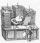The office was attended by 23 people. 9 of them completed the questionnaire. What is the percentage of all people?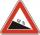Road sign informs the gradient is 10.3%. Calculate the angle which average decreases.
• ShotsDetermine the percentage rate of keeper interventions if from 32 shots doesn't caught four shots.
• Identity123456789 = 100 Use only three plus or minus characters to correct previous identity/equation.
• PopulationWhat is the population of the city with 3% annual growth, if in 10 years the city will have 60,000 residents?
• CirclesHow many different circles is determined by 9 points at the plane, if 6 of them lie in a straight line?
• Permill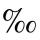How many permill is 978 from 84370?
• Wage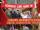Increasing the minimum wage while maintaining the other parameters of the system, unemployment will be:
• Car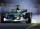Car is going 95 km per hour. How long distance goes in 1 minute?
• Equation algebraogram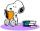Solve the equation: oco + ivo = cita How much has the task of solutions?
• Cancel fractionsCompress the expression of factorial: (n+6)!/(n+4)!-n!/(n-2)!
• Reciprocal equationDetermine the root of the equation: 9/x-7/x=1
• Ratio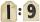Unfold number 1963 in the ratio 9:2:2.
• Proportional relationship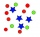The ordered pairs (6,24) and (1, s) represent a proportional relationship. Find the value of s.
• Eggs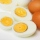One egg boiled in 10 minutes, how long will it take to boil ten eggs at a time?
• One threeWe throw two dice. What is the probability that max one three falls?
• Ratio - proportion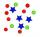Reduce the number 16 in proportion 3:2 5:4 11:8
• SlopeWhat is the slope of the line defined by the equation -2x +3y = -1 ?

Do you have an interesting mathematical word problem that you can't solve it? Submit a math problem, and we can try to solve it.

We will send a solution to your e-mail address. Solved examples are also published here. Please enter the e-mail correctly and check whether you don't have a full mailbox.

Please do not submit problems from current active competitions such as Mathematical Olympiad, correspondence seminars etc...